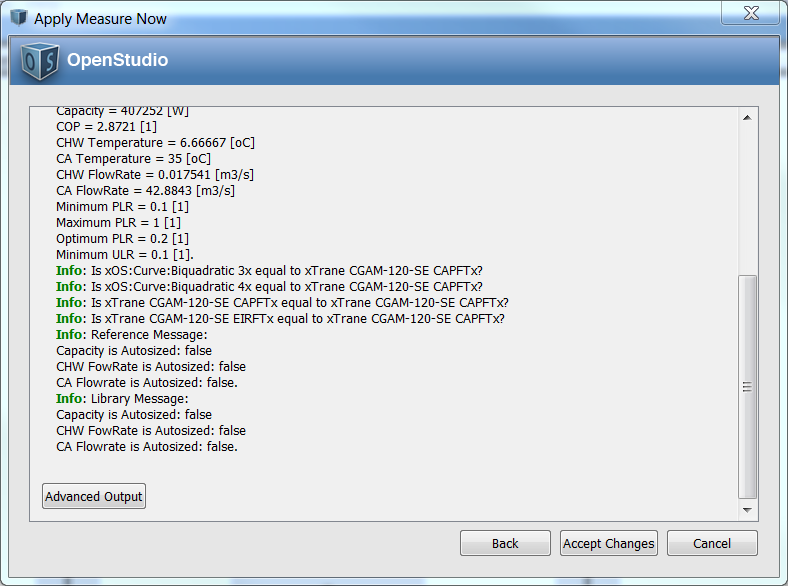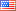Question-and-Answer Resource for the Building Energy Modeling Community
Get started with the Help page

# Revision history [back]

### replace, not clone, a curve?

I was able to use an existing code snippet to create (or clone, if it already exists) a particular curve. However, if a curve of that name (say "Curve") already exists, then this will add another curve (say "Curve 1"), and each time this is called, there will be another (say "Curve 2", etc.) - the meaning of clone, I suppose.

 library_Factor2 = curve.get.to_ModelObject.get.clone(model).to_CurveBiquadratic.get 

Is there a way to replace (or first delete and then create) that curve, so that I will only ever have a curve named "Curve" in the model? Something like (hopefully it is that easy) the following, perhaps?

 library_Factor2 = curve.get.to_ModelObject.get.replace(model).to_CurveBiquadratic.get 

Where the replace function would either create or replace, not create or clone?

### replace, not clone, a curve?

I was able to use an existing code snippet to create (or clone, if it already exists) a particular curve. However, if a curve of that name (say "Curve") already exists, then this will add another curve (say "Curve 1"), and each time this is called, there will be another (say "Curve 2", etc.) - the meaning of clone, I suppose.

 library_Factor2 = curve.get.to_ModelObject.get.clone(model).to_CurveBiquadratic.get 

Is there a way to replace (or first delete and then create) that curve, so that I will only ever have a curve named "Curve" in the model? Something like (hopefully it is that easy) the following, perhaps?

 library_Factor2 = curve.get.to_ModelObject.get.replace(model).to_CurveBiquadratic.get 

Where the replace function would either create or replace, not create or clone?

<<< EDIT <<<

OK, some progress, thanks. I did the following:

 model_Curves = model.getCurveBiquadratics model_Curves.each do |model_Curve| runner.registerInfo("Is x#{model_Curve.name}x equal to x#{curve_name}x?") if model_Curve.name == curve_name runner.registerInfo("Yes, x#{model_Curve.name}x is equal to x#{curve_name}x!") break end end 

Unfortunately, it does not quite work, as the result in the image below shows.It seems to me that "Trane CGAM-120-SE CAPFT" should have been caught and entered the above if comparison, but it does not seem to? Also, should I be able to enter the if comparison, I could then simply use

 model_Curve.remove 

Is that correct?

### replace, not clone, a curve?

I was able to use an existing code snippet to create (or clone, if it already exists) a particular curve. However, if a curve of that name (say "Curve") already exists, then this will add another curve (say "Curve 1"), and each time this is called, there will be another (say "Curve 2", etc.) - the meaning of clone, I suppose.

 library_Factor2 = curve.get.to_ModelObject.get.clone(model).to_CurveBiquadratic.get 

Is there a way to replace (or first delete and then create) that curve, so that I will only ever have a curve named "Curve" in the model? Something like (hopefully it is that easy) the following, perhaps?

 library_Factor2 = curve.get.to_ModelObject.get.replace(model).to_CurveBiquadratic.get 

Where the replace function would either create or replace, not create or clone?

<<< EDIT <<<

OK, some progress, thanks. I did the following:

 model_Curves = model.getCurveBiquadratics model.getCurveBiquadratics model_Curves.each do |model_Curve| |model_Curve| runner.registerInfo("Is x#{model_Curve.name}x equal to x#{curve_name}x?") x#{curve_name}x?") if model_Curve.name == curve_name curve_name runner.registerInfo("Yes, x#{model_Curve.name}x is equal to x#{curve_name}x!") break end x#{curve_name}x!") break end  end

Unfortunately, it does not quite work, as the result in the image below shows.It seems to me that "Trane CGAM-120-SE CAPFT" should have been caught and entered the above if comparison, but it does not seem to? Also, should I be able to enter the if comparison, I could then simply use

 model_Curve.remove model_Curve.remove

Is that correct?

### replace, not clone, a curve?

I was able to use an existing code snippet to create (or clone, if it already exists) a particular curve. However, if a curve of that name (say "Curve") already exists, then this will add another curve (say "Curve 1"), and each time this is called, there will be another (say "Curve 2", etc.) - the meaning of clone, I suppose.

 library_Factor2 = curve.get.to_ModelObject.get.clone(model).to_CurveBiquadratic.get 

Is there a way to replace (or first delete and then create) that curve, so that I will only ever have a curve named "Curve" in the model? Something like (hopefully it is that easy) the following, perhaps?

 library_Factor2 = curve.get.to_ModelObject.get.replace(model).to_CurveBiquadratic.get 

Where the replace function would either create or replace, not create or clone?

<<< EDIT <<<

OK, some progress, thanks. I did the following:

model_Curves = model.getCurveBiquadratics
model_Curves.each do |model_Curve|
runner.registerInfo("Is x#{model_Curve.name}x equal to x#{curve_name}x?")
if model_Curve.name == curve_name
runner.registerInfo("Yes, x#{model_Curve.name}x is equal to x#{curve_name}x!")
break
end
endend


Unfortunately, it does not quite work, as the result in the image below shows.It seems to me that "Trane CGAM-120-SE CAPFT" should have been caught and entered the above if comparison, but it does not seem to? Also, should I be able to enter the if comparison, I could then simply use

model_Curve.remove

model_Curve.remove


Is that correct?5 retagged__AmirRoth__3989 ●4 ●14 http://bleedinggreenna...

### replace, not clone, a curve?

I was able to use an existing code snippet to create (or clone, if it already exists) a particular curve. However, if a curve of that name (say "Curve") already exists, then this will add another curve (say "Curve 1"), and each time this is called, there will be another (say "Curve 2", etc.) - the meaning of clone, I suppose.

 library_Factor2 = curve.get.to_ModelObject.get.clone(model).to_CurveBiquadratic.get 

Is there a way to replace (or first delete and then create) that curve, so that I will only ever have a curve named "Curve" in the model? Something like (hopefully it is that easy) the following, perhaps?

 library_Factor2 = curve.get.to_ModelObject.get.replace(model).to_CurveBiquadratic.get 

Where the replace function would either create or replace, not create or clone?

<<< EDIT <<<

OK, some progress, thanks. I did the following:

model_Curves = model.getCurveBiquadratics
model_Curves.each do |model_Curve|
runner.registerInfo("Is x#{model_Curve.name}x equal to x#{curve_name}x?")
if model_Curve.name == curve_name
runner.registerInfo("Yes, x#{model_Curve.name}x is equal to x#{curve_name}x!")
break
end
end


Unfortunately, it does not quite work, as the result in the image below shows.It seems to me that "Trane CGAM-120-SE CAPFT" should have been caught and entered the above if comparison, but it does not seem to? Also, should I be able to enter the if comparison, I could then simply use

model_Curve.remove


Is that correct?